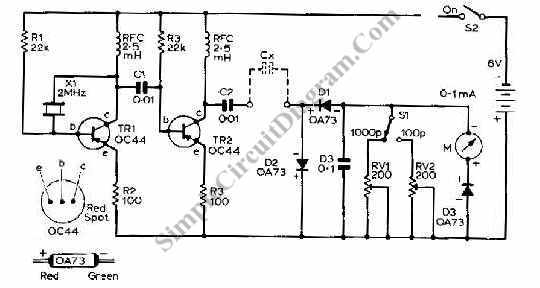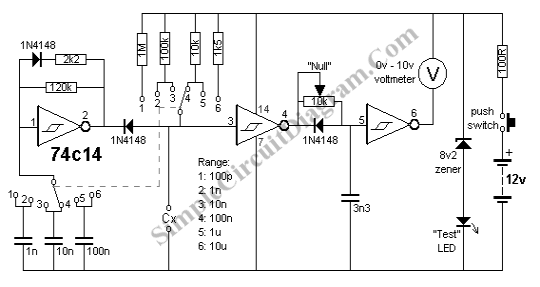## Transistor Capacitance Meter CircuitThis is a circuit that can be used to meter the capacitance. This circuit is similar to previous meter circuit. It has a little difference, this circuit uses transistors rather than logic gates. Here is the circuit: This circuit uses pnp transistor. if we can’t find exact type as shown in the circuit, we can use almost any pnp transistor […]